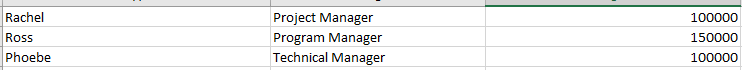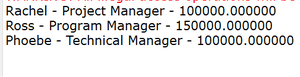# Java Program to Extract Content from a Excel sheet

• Last Updated : 09 Nov, 2021

Spreadsheets are the easier way to represent table like data and can give a visual representation of data in tabular format. In this article, let us see how to extract the contents of the Excel sheet via java. Here there arise two cases where Maven is considered in the program or not. Discussing both of them as a prerequisite for a better understanding of the program.

Apache POI API basics are crucial before moving ahead, so the two main prefixes are required when working with Apache POI are as follows:

1. HSSF: denotes the API is for working with Excel 2003 and earlier.
2. XSSF: denotes the API is for working with Excel 2007 and later.

Following 4 interfaces are important and essential to go through

• Workbook: High-level representation of an Excel workbook. HSSFWorkbook and XSSFWorkbook.
• Sheet: High-level representation of an Excel worksheet. Typical implementing classes are HSSFSheetand XSSFSheet.
• Row: Highlevel representation of a row in a spreadsheet. HSSFRow and XSSFRow are two concrete classes.
• Cell: High-level representation of a cell in a row. HSSFCell and XSSFCell are the typical implementing classes.

Case 1: Maven java project where dependencies are as follows

• All Maven projects will have pom.xml as the main file.
• There we need to add the dependencies.
• pom.xml file contents Excel formats do differ a bit as shown below:
• It is recommended to specify the latest version. (Maven project example used here is 3.11)

For Excel 2003 format

```<dependency>
<groupId>org.apache.poi</groupId>
<artifactId>poi</artifactId>
<version>VERSION</version>
</dependency>```

For Excel 2007 format

```<dependency>
<groupId>org.apache.poi</groupId>
<artifactId>poi-ooxml</artifactId>
<version>VERSION</version>
</dependency>```

Case 2: Non-Maven Java project.

In order to meet the requirements there arises an urgent need to add the jar files in the build path in order to extract the contents. For this, download the latest release of the Apache POI library.

To extract the contents from

Excel 2003 format

`poi-VERSION.jar is enough.`

Excel 2007 format :

```poi-ooxml-VERSION.jar
poi-ooxml-schemas-VERSION.jar
xmlbeans-VERSION.jar```

Procedure: Reading from Excel file using Apache POI with examples. The goal is to read the contents from the given Excel file and display the contents of the Excel file in the “output” window.

Step 1: Here we are using the POJO class which has an equal number of fields as given in the attached Excel file. Excel file got 3 columns and hence 3 fields are there in the POJO class. Sample Excel file contents are as shown below. It is always nicer to have a POJO(Plain old java object) class for these kinds of operations. As there are 3 column values and the details are related to an employee, let us have an employee class.

Sample input image:Empname, EmpDesignation, Salary are the columns

Example:

## Java

 `// Java Program in which a Class is declared and``// its methods are defined` `// Class``class` `Employee {` `    ``// Member variable of Employee Class``    ``// Name, Designation and Salary``    ``private` `String employeeName;``    ``private` `String employeeDesignation;``    ``private` `double` `salary;` `    ``// Constructor of Employee class``    ``public` `Employee() {}` `    ``// Method 1``    ``public` `String toString()``    ``{``        ``return` `String.format(``"%s - %s - %f"``, employeeName,``                             ``employeeDesignation, salary);``    ``}` `    ``// method 2``    ``// To get name of an employee``    ``public` `String getEmployeeName()``    ``{` `        ``// Return the name of the employee``        ``return` `employeeName;``    ``}` `    ``// Method - 3``    ``// To set employee name``    ``public` `void` `setEmployeeName(String employeeName)``    ``{` `        ``// This keyword refer to the current``        ``// method or constructor itself``        ``// Hence, same employee name can be set``        ``// through this method``        ``this``.employeeName = employeeName;``    ``}` `    ``// Method - 4``    ``// To get already assigned designation of``    ``// the employee over which method is invoked``    ``public` `String getEmployeeDesignation()``    ``{` `        ``// Return the designation of the employee``        ``// over which the function is called``        ``return` `employeeDesignation;``    ``}` `    ``// Method - 5``    ``// To assign a designation to an employee``    ``public` `void``    ``setEmployeeDesignation(String employeeDesignation)``    ``{` `        ``// This keyword refer to the current``        ``// method or constructor itself``        ``this``.employeeDesignation = employeeDesignation;``    ``}` `    ``// Method - 6``    ``// To get salary of an employee``    ``public` `double` `getSalary()``    ``{` `        ``// Return the salary of the employee for which``        ``// the function is invoked``        ``return` `salary;``    ``}` `    ``// Method - 7``    ``// To set salary of the existing employee with``    ``// assigned name and designation``    ``public` `void` `setSalary(``double` `salary)``    ``{``        ``this``.salary = salary;``    ``}``}`

Step 2: Depends on different data types like String, Number(it fits for integer, double, float, etc), Boolean, we need to have a method to get the cell values of Excel

Example:

## Java

 `// Java Program to get the cell value``// of the corresponding cells` `// Method``// To get the cell value``private` `Object getCellValue(Cell cell)``{` `    ``// Now either do-while or switch can be used``    ``// to display menu/user's choice` `    ``// Switch case is used here for illustration``    ``// Switch case to get the users choice``    ``switch` `(cell.getCellType()) {` `        ``// Case 1``        ``// If cell contents are string``    ``case` `Cell.CELL_TYPE_STRING:``        ``return` `cell.getStringCellValue();` `        ``// Case 2``        ``// If cell contents are Boolean``    ``case` `Cell.CELL_TYPE_BOOLEAN:``        ``return` `cell.getBooleanCellValue();` `        ``// Case 3``        ``// If cell contents are Numeric which includes``        ``// int, float , double etc``    ``case` `Cell.CELL_TYPE_NUMERIC:``        ``return` `cell.getNumericCellValue();``    ``}` `    ``// Case 4``    ``// Default case``    ``// If cell contents are neither``    ``// string nor Boolean nor Numeric,``    ``// simply nothing is returned``    ``return` `null``;``}`

Step 3:Method to extract Excel file contents. We need to specify the location of the file correctly. otherwise, it will end up with IOException

Example:

## Java

 `// Java Program to get the Excel file name``// as an argument` `public` `List``readDataFromExcelFile(String excelFilePath)``    ``throws` `IOException``{``    ``// Creating an List object of Employee type``    ``// Note: User defined type``    ``List listEmployees``        ``= ``new` `ArrayList();` `    ``FileInputStream inputStream``        ``= ``new` `FileInputStream(``new` `File(excelFilePath));` `    ``// As used 'xlsx' file is used so XSSFWorkbook will be``    ``// used``    ``Workbook workbook = ``new` `XSSFWorkbook(inputStream);` `    ``// Read the first sheet and if the contents are in``    ``// different sheets specifying the correct index``    ``Sheet firstSheet = workbook.getSheetAt(``0``);` `    ``// Iterators to traverse over``    ``Iterator iterator = firstSheet.iterator();` `    ``// Condition check using hasNext() method which holds``    ``// true till there is single element remaining in List` `    ``while` `(iterator.hasNext()) {``        ``// Get a row in sheet``        ``Row nextRow = iterator.next();``        ``// This is for a Row's cells``        ``Iterator cellIterator``            ``= nextRow.cellIterator();``        ``// We are taking Employee as reference.``        ``Employee emp = ``new` `Employee();``        ``// Iterate over the cells``        ``while` `(cellIterator.hasNext()) {``            ``Cell nextCell = cellIterator.next();` `            ``// Switch case variable to``            ``// get the columnIndex``            ``int` `columnIndex = nextCell.getColumnIndex();` `            ``// Depends upon the cell contents we need to``            ``// typecast` `            ``// Switch-case``            ``switch` `(columnIndex) {` `                ``// Case 1``            ``case` `0``:``                ``// First column is alpha and hence``                ``// it is typecasted to String``                ``emp.setEmployeeName(``                    ``(String)getCellValue(nextCell));``                ``// Break keyword to directly terminate``                ``// if this case is hit``                ``break``;` `                ``// Case 2``            ``case` `1``:``                ``// Second  column is alpha and hence``                ``// it is typecasted to String``                ``emp.setEmployeeDesignation(``                    ``(String)getCellValue(nextCell));``                ``// Break keyword to directly terminate``                ``// if this case is hit``                ``break``;` `                ``// Case 3``            ``case` `2``:``                ``// Third  column is double value and``                ``// hence it is typecasted to Double``                ``emp.setSalary(``                    ``(Double)getCellValue(nextCell));``                ``break``;` `                ``// Note: If additional cells are present``                ``// then``                ``// they should be specified further down,``                ``// and POJO class should accommodate those``                ``// cell values``            ``}``        ``}``        ``// Adding up to the list``        ``listEmployees.add(emp);``    ``}` `    ``// Closing the workbook and inputstream``    ``// as it free up the space in memory``    ``workbook.close();``    ``inputStream.close();` `    ``// Return all the employees present in List``    ``// object of Employee type``    ``return` `listEmployees;``}`

Step 4: Integrating the concepts from Step 1 to Step 3 in the Main program

## Java

 `// Main driver method``public` `static` `void` `main(String[] args)``{``    ``// Detecting the file type``    ``GetContentFromExcelSheets getContentFromExcelSheets``        ``= ``new` `GetContentFromExcelSheets();``    ``// Creating an List object of Employee type``    ``// in main() method``    ``List extractedEmployeeData``        ``= ``new` `ArrayList();` `    ``// Try block to check if any exception/s occurs``    ``try` `{``        ``// excelFileContents.xlsx location need to be``        ``// specified correctly or else IOException will be``        ``// thrown. If file is available in that location, it``        ``// gets the data and stored in a list variable``        ``extractedEmployeeData``            ``= getContentFromExcelSheets``                  ``.readDataFromExcelFile(``                      ``"excelFileContents.xlsx"``);``    ``}` `    ``// Catch block to handle the exceptions if occurred``    ``catch` `(IOException e) {` `        ``// Print the line number and exception``        ``// in the program``        ``e.printStackTrace();``    ``}` `    ``// As there are possibility of data in multiple cells,``    ``// it is always a good approach to follow a POJO pattern``    ``// and get a row value in specified POJO As all data is``    ``// collected in a list, we can iterate and display as``    ``// below``    ``for` `(``int` `i = ``0``; i < extractedEmployeeData.size(); i++) {` `        ``// Print and display the employees data to the``        ``//  console using toString() method to the user``        ``System.out.println(``            ``extractedEmployeeData.get(i).toString());``    ``}``}`

Output: For our example, we have 3 rows of data onlyImplementation:

Example:

## Java

 `// Java Program to Extract Content from a Excel sheet` `// As we are reading the excel file, java.io package is``// compulsorily required``import` `java.io.File;``import` `java.io.FileInputStream;``import` `java.io.IOException;``import` `java.util.ArrayList;``import` `java.util.Iterator;``import` `java.util.List;` `// Below imports are required to access Apache POI``// The usermodel package maps HSSF low level structures to``// familiar workbook/sheet model``// org.apache.poi.hssf.usermodel``// But we are using higher excel formats hence,``// org.apache.poi.ss.usermodel is used To determine the type``// of cell content``import` `org.apache.poi.ss.usermodel.Cell;` `// each and every row of excel is taken and stored in this``// row format``import` `org.apache.poi.ss.usermodel.Row;` `// excel sheet is read in this sheet format``import` `org.apache.poi.ss.usermodel.Sheet;` `// excel Workbook is read in this Workbook format``import` `org.apache.poi.ss.usermodel.Workbook;` `// XSSFWorkbook denotes the API is for working with Excel``// 2007 and later.``import` `org.apache.poi.xssf.usermodel.XSSFWorkbook;` `// POJO class having 3 fields matching with the given excel``// file``class` `Employee {``    ``private` `String employeeName;``    ``private` `String employeeDesignation;``    ``private` `double` `salary;``    ``// All 3 fields getter, setter methods should be there``    ``public` `Employee() {}` `    ``public` `String toString()``    ``{``        ``return` `String.format(``"%s - %s - %f"``, employeeName,``                             ``employeeDesignation, salary);``    ``}` `    ``public` `String getEmployeeName() { ``return` `employeeName; }` `    ``public` `void` `setEmployeeName(String employeeName)``    ``{``        ``this``.employeeName = employeeName;``    ``}` `    ``public` `String getEmployeeDesignation()``    ``{``        ``return` `employeeDesignation;``    ``}` `    ``public` `void``    ``setEmployeeDesignation(String employeeDesignation)``    ``{``        ``this``.employeeDesignation = employeeDesignation;``    ``}` `    ``public` `double` `getSalary() { ``return` `salary; }` `    ``public` `void` `setSalary(``double` `d) { ``this``.salary = d; }``}``// class to assign the cell value once it is getting done to``// read from excel sheet It can be String/Boolean/Numeric``public` `class` `GetContentFromExcelSheets {``    ``private` `Object getCellValue(Cell cell)``    ``{``        ``switch` `(cell.getCellType()) {``        ``case` `Cell.CELL_TYPE_STRING:``            ``return` `cell.getStringCellValue();` `        ``case` `Cell.CELL_TYPE_BOOLEAN:``            ``return` `cell.getBooleanCellValue();` `        ``case` `Cell.CELL_TYPE_NUMERIC:``            ``return` `cell.getNumericCellValue();``        ``}` `        ``return` `null``;``    ``}``    ``// Read the excel sheet contents and get the contents in``    ``// a list``    ``public` `List``    ``readBooksFromExcelFile(String excelFilePath)``        ``throws` `IOException``    ``{``        ``List listEmployees``            ``= ``new` `ArrayList();``        ``FileInputStream inputStream``            ``= ``new` `FileInputStream(``new` `File(excelFilePath));` `        ``Workbook workbook = ``new` `XSSFWorkbook(inputStream);``        ``Sheet firstSheet = workbook.getSheetAt(``0``);``        ``Iterator iterator = firstSheet.iterator();` `        ``while` `(iterator.hasNext()) {``            ``Row nextRow = iterator.next();``            ``Iterator cellIterator``                ``= nextRow.cellIterator();``            ``Employee emp = ``new` `Employee();` `            ``while` `(cellIterator.hasNext()) {``                ``Cell nextCell = cellIterator.next();``                ``int` `columnIndex = nextCell.getColumnIndex();` `                ``switch` `(columnIndex) {``                ``case` `1``:``                    ``emp.setEmployeeName(``                        ``(String)getCellValue(nextCell));``                    ``break``;``                ``case` `2``:``                    ``emp.setEmployeeDesignation(``                        ``(String)getCellValue(nextCell));``                    ``break``;``                ``case` `3``:``                    ``emp.setSalary(Double.valueOf(``                        ``(String)getCellValue(nextCell)));``                    ``break``;``                ``}``            ``}``            ``listEmployees.add(emp);``        ``}` `        ``((FileInputStream)workbook).close();``        ``inputStream.close();` `        ``return` `listEmployees;``    ``}` `    ``// Main program``    ``public` `static` `void` `main(String[] args)``    ``{``        ``// detecting the file type``        ``GetContentFromExcelSheets getContentFromExcelSheets``            ``= ``new` `GetContentFromExcelSheets();``        ``List extractedEmployeeData``            ``= ``new` `ArrayList();``        ``try` `{``            ``extractedEmployeeData``                ``= getContentFromExcelSheets``                      ``.readBooksFromExcelFile(``                          ``"excelFileContents.xlsx"``);``        ``}``        ``catch` `(IOException e) {``            ``// TODO Auto-generated catch block``            ``e.printStackTrace();``        ``}``        ``System.out.println(extractedEmployeeData);``    ``}``}`

Conclusion : Apache POI provides a nicer implementation to extract Excel file contents. In coding, according to the availability of data in the number of cells, we need to have POJO class attributes and also we need to specify coll data in the “readDataFromExcelFile” method. We can format Double data as per our requirement also.

My Personal Notes arrow_drop_up